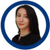# QlikView App Dev

Discussion Board for collaboration related to QlikView App Development.

cancel
Showing results for
Did you mean:Partner - Contributor

## Nested set analysisI want to show the sales from the 20 first countries which sale horror movies, but I don't know how to anidate the function with set analysis to the set analysis.

Sum({1<Genero={"*Horror*"},Pais={"\$(=rank(sum({1<Genero={"*Horror*"}>}Sales),1,1))<=20"}>}Sales)

i though with the dollar-sign expansion, but still doesn't works.

1 Solution

Accepted SolutionsMVP

May be this

`Sum({1<Genero = {"*Horror*"}, Pais = {"=Rank(Sum({1<Genero = {[*Horror*]}>} Sales), 1, 1)) <= 20"}>} Sales)``Sum({1<Genero = {"*Horror*"}, Pais = {"=Rank(Sum({1<Genero = {[*Horror*]}>} Sales), 1, 1)) <= 20"}>} Sales)`